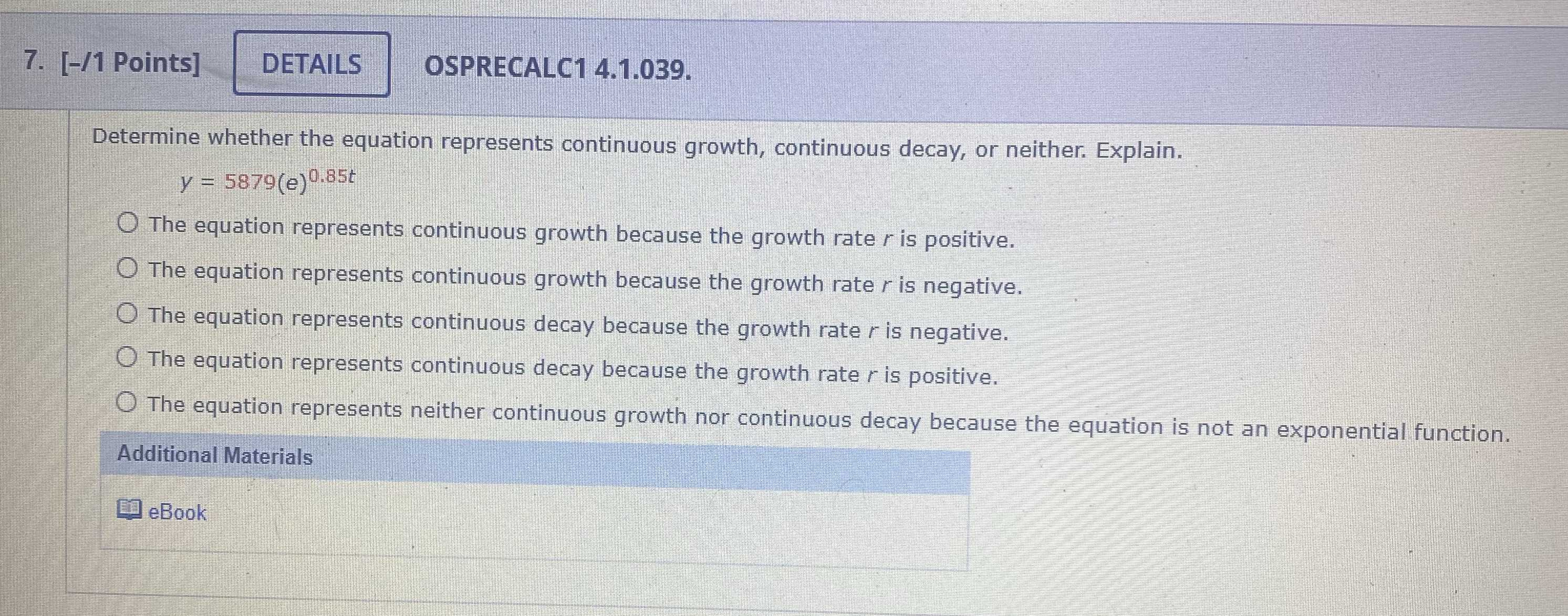### ¿Todavía tienes preguntas de matemáticas?

Pregunte a nuestros tutores expertos
Algebra
Pregunta7. Determine whether the equation represents continuous growth, continuous decay, or neither. Explain. $$y = 5879 ( e ) ^ { 0.85 t }$$

The equation represents continuous growth because the growth rate $$r$$ is positive.

The equation represents continuous growth because the growth rate $$r$$ is negative.

The equation represents continuous decay because the growth rate $$r$$ is negative.

The equation represents continuous decay because the growth rate $$r$$ is positive.

The equation represents neither continuous growth nor continuous decay because the equation is not an exponential function.